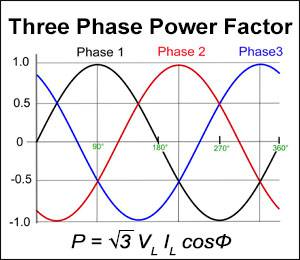# Three Phase Power Factor Calculator

This calculator determines the value for a variable in the three phase power factor. If Power Factor needs to be determined, leave the input field blank and fill in the other fields.

Output values are rounded to the second decimal place.

Out of the two formulas below, the line voltage, line current version is the one that is frequently used.

Three Phase Power Factor Formula

P = √3*V*I*cosθ

V=Line Voltage, I=Line Current, P = Power(W), cosθ = Power Factor

P = 3*V*I*cosθ

V=Phase Voltage, I=Phase Current, P = Power(W), cosθ = Power Factor### Input

Power:

Voltage:

Current:

Power Factor:

Line Voltage, Line Current
Phase Voltage, Phase Current

Leave one field blank.